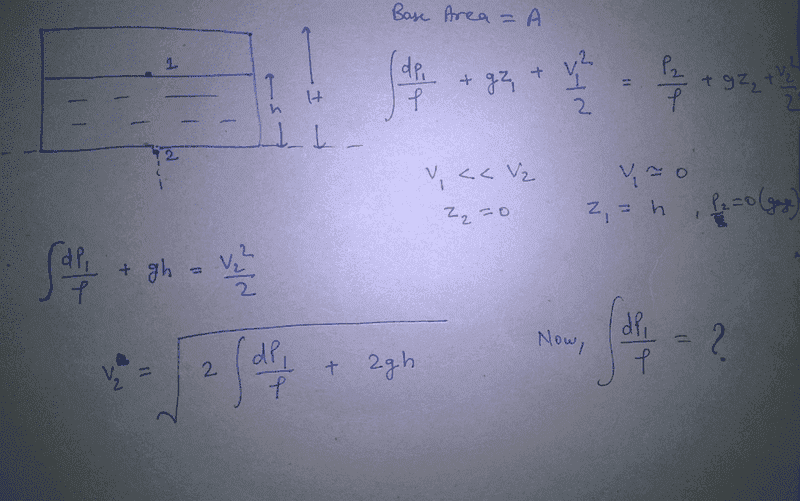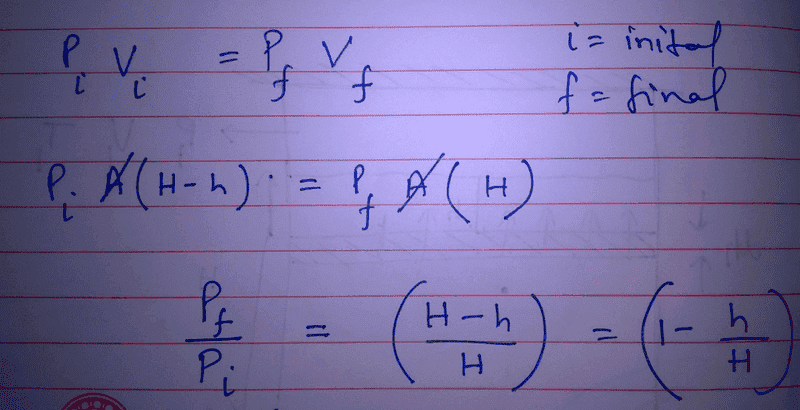# Velocity of liquid from bottom pinhole of closed container

What I know: Below link is about Torricelli's law. Velocity of liquid coming out of bottom of the tank i.e comes after using Bernoulli's equation square root of (2*g*h*) where "h" is height of fluid in the container and "g" is acceleration due to gravity.

https://en.wikipedia.org/wiki/Torricelli's_law

Doubt: All the time problem was given with the case of open container. That means gauge pressure is zero on the top of the surface of liquid and at bottom of the tank. Now this is the case with tank is with lid closed. Now what I think; we cannot neglect the pressure term in the Bernoull's equation because it would be the function of the height of fluid being reduced. As tank is being emptied; the density of air above the liquid is reducing so the pressure.
Then another doubt comes what if there is increase in the rate of evaporation and maintaining the density of air above liquid surface.
Pls help me to find out the expression of outlet velocity; I know it would be decreasing with the passage of time.

https://en.wikipedia.org/wiki/Torricelli's_law

jbriggs444
Homework Helper
How about starting with a simple version of the problem?

Ignore evaporation and assume a vapor pressure of zero. Assume that the air behaves as an ideal gas. Assume isothermal expansion. Assume that the tube/tank is large compared to the pinhole. Assume a vertical tube/tank with a uniform cross section. Pick a tube/tank height and an initial water height in the tube/tank.

Can you write a differential equation that relates the flow rate and the volume of water remaining in the tube/tankjbriggs444
Homework Helper
Ugly hand-written equations...

So you have a column of cross-section A, total height H and fluid height h. And you have a bunch of undefined variables and no resulting equation.

What was it that you are trying to express?

ok let me correct myself;

Lets apply bernoulli's equation for the starting situation....> P1 (pressure at the top of liquid) P2 (=0 at pinhole) V1(=0 velocity of fluid on the top of liquid layer) V2(pinhole fluid velocity), h(elevation difference)

After applying bernoulli's what we get;

#### Attachments

But here P1 is the function of rho(density)

jbriggs444
Homework Helper
But here P1 is the function of rho(density)
Where did P2 go?

Where did P2 go?
I am considering P1 as gauge pressure that's why P2 became zero

jbriggs444
Homework Helper
OK, so you have ##v_2 = \sqrt{2\frac{p_1}{\rho} + 2gh}##
We need to do some work on that equation. It has too many variables. We need to reduce it to one variable and a bunch of constants. If you look at post #2 I suggested using volume of fluid remaining in the tank as the single variable. Call that variable V.

##\rho## and ##g## are constants. Those are fine.
##p_1## can be expressed as a function of V, ambient absolute pressure, the initial height of fluid in the column and the total height of fluid in the column.
The current height of fluid in the column can be expressed as a function of V.

Start with those substitutions and we'll have most of the desired differential equation. Think about what you can do with ##v_2## to complete the job.

##\rho## and ##g## are constants. Those are fine.
##p_1## can be expressed as a function of V, ambient absolute pressure, the initial height of fluid in the column and the total height of fluid in the column.

Why density is assumed to be constant? It is changing as we considered as isothermal expansion

Last edited:jbriggs444
Homework Helper
Why density is assumed to be constant? It is changing as we considered as isothermal expansion
Because we want a problem that is simple enough to solve. The variation of the density of an ideal liquid with respect to pressure is ideally zero anyway.

I see that you have done some work on the head pressure. However, your answer is not in the form I suggested. In the formulation we are after, h is a constant, not a variable. It is the initial height of the liquid. The variable we want to use is V, the volume of fluid remaining. The immediate goal is to express head pressure (##p_1##) as a function of V.

Once you have ##p_1## as a function of V, substitute that into the equation for ##v_2##. Be careful. You have shifted between gauge and absolute pressure without telling anyone. That's the second time you pulled that trick.

Last edited:
I misinterpreted the density as of air; I forgot it was of liquid. I admitted my mistake from shifting of gauge to absolute pressure. But I am not understanding how I could make p1 as a function of V. I am feeling helpless.

jbriggs444
Homework Helper
I misinterpreted the density as of air; I forgot it was of liquid. I admitted my mistake from shifting of gauge to absolute pressure. But I am not understanding how I could make p1 as a function of V. I am feeling helpless.
You know the starting volume of air in the headspace, yes?
You know the starting pressure of air in the headspace, yes?
You know the total volume of the tank, yes?
If you know the volume of liquid remaining, then know the current volume of the headspace.
You have been given the assumption of isothermal expansion.
What is the pressure in the headspace?

•Ravi Singh choudhary
Starting volume of air in the headspace=A(H-h)
Starting pressure of air in the headspace=P(atm)
Volume of the tank=AH

Volume of liquid remaining=Ah-Ax; where x is depth of fluid remaining.

Current pressure=P2

(P2)*(AH-Ah+Ax)=P(atm)*A(H-h); isothermal expansion.

here P2 is dependent on the variable "x"; all other are constants.

jbriggs444
Homework Helper
Volume of liquid remaining=Ah-Ax; where x is depth of fluid remaining.
So by "depth" of fluid, you mean the amount by which its current surface height is below its starting surface height? That's fine. We can do our differential equation in terms of x rather than in terms of V.

Let's write that last equation neatly in LaTeX and put ##P_2## along on the left hand side. Dividing by ##AH-Ah+Ax## we get

##P_2=\frac{AP_{atm}(H-h)}{A(H-h+x)}##
## = P_{atm} \frac{H-h}{H-h+x}##

To embed TeX within a posting, you can use two back to back pound signs both in front and behind the formula. # # [formula] # #. This is called embedded TeX or itex for short. The double pound signs are a shorthand and can be replaced with the tags [ itex ] and [ /itex ] (leave out the blanks to make them actually work)

For example: # # pound signs # # yields ##pound signs## and [ itex ] tags [ /itex ] yields $tags$

Subscripts are keyed in with underscores, e.g. P_2 renders as ##P_2##.
Superscripts are keyed in with carets, e.g. x^2 renders as ##x^2##
You can group things with curly braces. So P_{atm} renders as ##P_{atm}## while P_atm renders as ##P_atm##
Fractions are done with \frac{top}{bottom} which renders as ##\frac{top}{bottom}##

If you want an entire line formatted using TeX, you can use [ tex ] and [ /tex ]. E.g. $$P_2 = \frac{AP_{atm}(H-h)}{A(H-h+x)}$$
Now, can you put this together and write the current flow velocity in terms of x using TeX? If you look at post #9, you'll find a good start.

Last edited:
•Ravi Singh choudhary
Leave the equations for the time being; let me rewind the all concepts used here.

Bernoulli's applied but making pressure term as variable. Gage pressure used first; so after finding pressure variation; P(atm) must be subtracted from the absolute pressure term.

Isothermal expansion; pressure of headspace became the function of the depth of fluid.

Anything else.

jbriggs444
Homework Helper
Bernoulli's applied but making pressure term as variable. Gage pressure used first; so after finding pressure variation; P(atm) must be subtracted from the absolute pressure term.

Isothermal expansion; pressure of headspace became the function of the depth of fluid.

Anything else.
You also have to account for the pressure from gravity based on the current liquid depth, of course.

You also have to account for the pressure from gravity based on the current liquid depth, of course.

yeah ok :) Thank you. It is one the question that is being asked in many scientist interviews of INDIA.

There is a new complication to the question; What if the container is full with water and covered and then pinhole is made.

What could happen??

jbriggs444
Homework Helper
There is a new complication to the question; What if the container is full with water and covered and then pinhole is made.
What do you think could happen?

What would be the initial pressure at the top of the container; I think it must be zero. So pressure will be independent of volume of fluid left in the container. Absolute pressure in the headspace that would be created in the meantime will always be zero.

Considering there is no phase change of liquid.

jbriggs444
Homework Helper
What would be the initial pressure at the top of the container; I think it must be zero. So pressure will be independent of volume of fluid left in the container. Absolute pressure in the headspace that would be created in the meantime will always be zero.

Considering there is no phase change of liquid.
If the liquid is water and the pressure at the top is zero, what would be the pressure in the water, one meter below the top? If the pinhole is located at that point, what would that mean for the direction of flow?

If the liquid is water and the pressure at the top is zero, what would be the pressure in the water, one meter below the top? If the pinhole is located at that point, what would that mean for the direction of flow?

Ok I got you. But Pressure at pinhole will be always atmospheric; as it is open to atmosphere. Yeah I know how pressure varies in water with depth i.e. Density of water multiplied by g and height of fluid from the surface.

Last edited:
jbriggs444
Homework Helper
Ok I got you. But Pressure at pinhole will be always atmospheric; as it is open to atmosphere. Yeah I know how pressure varies in water with depth i.e. Density of water multiplied by g and height of fluid from the surface.
I do not think that I have explained myself well. The pressure on the outside of the pinhole will be atmospheric. If there is a flow through the pinhole, the pressure on the inside will not be atmospheric. Something has to drive the flow after all.

But the point I was trying to make is different. If a water tank is 10 meters high then the weight of the water is enough that draining it from the bottom can leave a vacuum at the top. But if a water tank is only 1 meter high then there is not enough water to pull a vacuum. The water will not readily drain. At best it will "glug out". [If the term is unfamiliar, Google can explain, complete with physics-based explanations].

•Ravi Singh choudhary
I read about glug out in Google; kind of emptying of water bottle. When some air enters to the bottle; liquid comes out and the cycle repeats. But this is a pinhole how air could enter to the tank

jbriggs444
Homework Helper
But this is a pinhole how air could enter to the tank
I agree. Air does not enter the tank in the case of a single pinhole.

I agree. Air does not enter the tank in the case of a single pinhole.

That means somehow fluid flow will come to halt, when fluid is just of small head left. Only we would see falling of droplets of liquid.

jbriggs444